Prealgebra

# 9.4Use Properties of Rectangles, Triangles, and Trapezoids

Prealgebra9.4 Use Properties of Rectangles, Triangles, and Trapezoids

### Learning Objectives

By the end of this section, you will be able to:
• Understand linear, square, and cubic measure
• Use properties of rectangles
• Use properties of triangles
• Use properties of trapezoids

### Be Prepared 9.4

Before you get started, take this readiness quiz.

1. The length of a rectangle is $33$ less than the width. Let $ww$ represent the width. Write an expression for the length of the rectangle.
If you missed this problem, review Example 2.26.
2. Simplify: $12(6h).12(6h).$
If you missed this problem, review Example 7.7.
3. Simplify: $52(10.3−7.9).52(10.3−7.9).$
If you missed this problem, review Example 5.36.

In this section, we’ll continue working with geometry applications. We will add some more properties of triangles, and we’ll learn about the properties of rectangles and trapezoids.

### Understand Linear, Square, and Cubic Measure

When you measure your height or the length of a garden hose, you use a ruler or tape measure (Figure 9.13). A tape measure might remind you of a line—you use it for linear measure, which measures length. Inch, foot, yard, mile, centimeter and meter are units of linear measure.

Figure 9.13 This tape measure measures inches along the top and centimeters along the bottom.

When you want to know how much tile is needed to cover a floor, or the size of a wall to be painted, you need to know the area, a measure of the region needed to cover a surface. Area is measured is square units. We often use square inches, square feet, square centimeters, or square miles to measure area. A square centimeter is a square that is one centimeter (cm) on each side. A square inch is a square that is one inch on each side (Figure 9.14).

Figure 9.14 Square measures have sides that are each $11$ unit in length.

Figure 9.15 shows a rectangular rug that is $22$ feet long by $33$ feet wide. Each square is $11$ foot wide by $11$ foot long, or $11$ square foot. The rug is made of $66$ squares. The area of the rug is$66$ square feet.

Figure 9.15 The rug contains six squares of 1 square foot each, so the total area of the rug is 6 square feet.

When you measure how much it takes to fill a container, such as the amount of gasoline that can fit in a tank, or the amount of medicine in a syringe, you are measuring volume. Volume is measured in cubic units such as cubic inches or cubic centimeters. When measuring the volume of a rectangular solid, you measure how many cubes fill the container. We often use cubic centimeters, cubic inches, and cubic feet. A cubic centimeter is a cube that measures one centimeter on each side, while a cubic inch is a cube that measures one inch on each side (Figure 9.16).

Figure 9.16 Cubic measures have sides that are 1 unit in length.

Suppose the cube in Figure 9.17 measures $33$ inches on each side and is cut on the lines shown. How many little cubes does it contain? If we were to take the big cube apart, we would find $2727$ little cubes, with each one measuring one inch on all sides. So each little cube has a volume of $11$ cubic inch, and the volume of the big cube is $2727$ cubic inches.

Figure 9.17 A cube that measures 3 inches on each side is made up of 27 one-inch cubes, or 27 cubic inches.

### Manipulative Mathematics

Doing the Manipulative Mathematics activity Visualizing Area and Perimeter will help you develop a better understanding of the difference between the area of a figure and its perimeter.

### Example 9.25

For each item, state whether you would use linear, square, or cubic measure:

1. amount of carpeting needed in a room

2. extension cord length

3. amount of sand in a sandbox

4. length of a curtain rod

5. amount of flour in a canister

6. size of the roof of a doghouse.

### Try It 9.49

Determine whether you would use linear, square, or cubic measure for each item.

amount of paint in a can height of a tree floor of your bedroom diameter of bike wheel size of a piece of sod amount of water in a swimming pool

### Try It 9.50

Determine whether you would use linear, square, or cubic measure for each item.

volume of a packing box size of patio amount of medicine in a syringe length of a piece of yarn size of housing lot height of a flagpole

Many geometry applications will involve finding the perimeter or the area of a figure. There are also many applications of perimeter and area in everyday life, so it is important to make sure you understand what they each mean.

Picture a room that needs new floor tiles. The tiles come in squares that are a foot on each side—one square foot. How many of those squares are needed to cover the floor? This is the area of the floor.

Next, think about putting new baseboard around the room, once the tiles have been laid. To figure out how many strips are needed, you must know the distance around the room. You would use a tape measure to measure the number of feet around the room. This distance is the perimeter.

### Perimeter and Area

The perimeter is a measure of the distance around a figure.

The area is a measure of the surface covered by a figure.

Figure 9.18 shows a square tile that is $11$ inch on each side. If an ant walked around the edge of the tile, it would walk $44$ inches. This distance is the perimeter of the tile.

Since the tile is a square that is $11$ inch on each side, its area is one square inch. The area of a shape is measured by determining how many square units cover the shape.

Figure 9.18 $Perimeter=4inchesArea=1square inchPerimeter=4inchesArea=1square inch$
When the ant walks completely around the tile on its edge, it is tracing the perimeter of the tile. The area of the tile is 1 square inch.

### Manipulative Mathematics

Doing the Manipulative Mathematics activity Measuring Area and Perimeter will help you develop a better understanding of how to measure the area and perimeter of a figure.

### Example 9.26

Each of two square tiles is $11$ square inch. Two tiles are shown together.

1. What is the perimeter of the figure?

2. What is the area?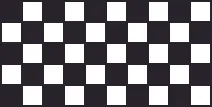### Try It 9.51

Find the perimeter and area of the figure:### Try It 9.52

Find the perimeter and area of the figure: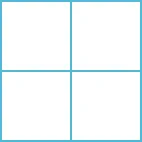### Use the Properties of Rectangles

A rectangle has four sides and four right angles. The opposite sides of a rectangle are the same length. We refer to one side of the rectangle as the length, $L,L,$ and the adjacent side as the width, $W.W.$ See Figure 9.19.

Figure 9.19 A rectangle has four sides, and four right angles. The sides are labeled L for length and W for width.

The perimeter, $P,P,$ of the rectangle is the distance around the rectangle. If you started at one corner and walked around the rectangle, you would walk $L+W+L+WL+W+L+W$ units, or two lengths and two widths. The perimeter then is

$P=L+W+L+WorP=2L+2WP=L+W+L+WorP=2L+2W$

What about the area of a rectangle? Remember the rectangular rug from the beginning of this section. It was $22$ feet long by $33$ feet wide, and its area was $66$ square feet. See Figure 9.20. Since $A=2⋅3,A=2⋅3,$ we see that the area, $A,A,$ is the length, $L,L,$ times the width, $W,W,$ so the area of a rectangle is $A=L⋅W.A=L⋅W.$

Figure 9.20 The area of this rectangular rug is $66$ square feet, its length times its width.

### Properties of Rectangles

• Rectangles have four sides and four right $(90°)(90°)$ angles.
• The lengths of opposite sides are equal.
• The perimeter, $P,P,$ of a rectangle is the sum of twice the length and twice the width. See Figure 9.19.
$P=2L+2WP=2L+2W$
• The area, $A,A,$ of a rectangle is the length times the width.
$A=L⋅WA=L⋅W$

For easy reference as we work the examples in this section, we will restate the Problem Solving Strategy for Geometry Applications here.

### How To

#### Use a Problem Solving Strategy for Geometry Applications

1. Step 1. Read the problem and make sure you understand all the words and ideas. Draw the figure and label it with the given information.
2. Step 2. Identify what you are looking for.
3. Step 3. Name what you are looking for. Choose a variable to represent that quantity.
4. Step 4. Translate into an equation by writing the appropriate formula or model for the situation. Substitute in the given information.
5. Step 5. Solve the equation using good algebra techniques.
6. Step 6. Check the answer in the problem and make sure it makes sense.
7. Step 7. Answer the question with a complete sentence.

### Example 9.27

The length of a rectangle is $3232$ meters and the width is $2020$ meters. Find the perimeter, and the area.

### Try It 9.53

The length of a rectangle is $120120$ yards and the width is $5050$ yards. Find the perimeter and the area.

### Try It 9.54

The length of a rectangle is $6262$ feet and the width is $4848$ feet. Find the perimeter and the area.

### Example 9.28

Find the length of a rectangle with perimeter $5050$ inches and width $1010$ inches.

### Try It 9.55

Find the length of a rectangle with a perimeter of $8080$ inches and width of $2525$ inches.

### Try It 9.56

Find the length of a rectangle with a perimeter of $3030$ yards and width of $66$ yards.

In the next example, the width is defined in terms of the length. We’ll wait to draw the figure until we write an expression for the width so that we can label one side with that expression.

### Example 9.29

The width of a rectangle is two inches less than the length. The perimeter is $5252$ inches. Find the length and width.

### Try It 9.57

The width of a rectangle is seven meters less than the length. The perimeter is $5858$ meters. Find the length and width.

### Try It 9.58

The length of a rectangle is eight feet more than the width. The perimeter is $6060$ feet. Find the length and width.

### Example 9.30

The length of a rectangle is four centimeters more than twice the width. The perimeter is $3232$ centimeters. Find the length and width.

### Try It 9.59

The length of a rectangle is eight more than twice the width. The perimeter is $6464$ feet. Find the length and width.

### Try It 9.60

The width of a rectangle is six less than twice the length. The perimeter is $1818$ centimeters. Find the length and width.

### Example 9.31

The area of a rectangular room is $168168$ square feet. The length is $1414$ feet. What is the width?

### Try It 9.61

The area of a rectangle is $598598$ square feet. The length is $2323$ feet. What is the width?

### Try It 9.62

The width of a rectangle is $2121$ meters. The area is $609609$ square meters. What is the length?

### Example 9.32

The perimeter of a rectangular swimming pool is $150150$ feet. The length is $1515$ feet more than the width. Find the length and width.

### Try It 9.63

The perimeter of a rectangular swimming pool is $200200$ feet. The length is $4040$ feet more than the width. Find the length and width.

### Try It 9.64

The length of a rectangular garden is $3030$ yards more than the width. The perimeter is $300300$ yards. Find the length and width.

### Use the Properties of Triangles

We now know how to find the area of a rectangle. We can use this fact to help us visualize the formula for the area of a triangle. In the rectangle in Figure 9.20, we’ve labeled the length $bb$ and the width $h,h,$ so it’s area is $bh.bh.$

Figure 9.21 The area of a rectangle is the base, $b,b,$ times the height, $h.h.$

We can divide this rectangle into two congruent triangles (Figure 9.22). Triangles that are congruent have identical side lengths and angles, and so their areas are equal. The area of each triangle is one-half the area of the rectangle, or $12bh.12bh.$ This example helps us see why the formula for the area of a triangle is $A=12bh.A=12bh.$

Figure 9.22 A rectangle can be divided into two triangles of equal area. The area of each triangle is one-half the area of the rectangle.

The formula for the area of a triangle is $A=12bh,A=12bh,$ where $bb$ is the base and $hh$ is the height.

To find the area of the triangle, you need to know its base and height. The base is the length of one side of the triangle, usually the side at the bottom. The height is the length of the line that connects the base to the opposite vertex, and makes a $90°90°$ angle with the base. Figure 9.23 shows three triangles with the base and height of each marked.

Figure 9.23 The height $hh$ of a triangle is the length of a line segment that connects the the base to the opposite vertex and makes a $90°90°$ angle with the base.

### Triangle Properties

For any triangle $ΔABC,ΔABC,$ the sum of the measures of the angles is $180°.180°.$

$m∠A+m∠B+m∠C=180°m∠A+m∠B+m∠C=180°$

The perimeter of a triangle is the sum of the lengths of the sides.

$P=a+b+cP=a+b+c$

The area of a triangle is one-half the base, $b,b,$ times the height, $h.h.$

$A=12bhA=12bh$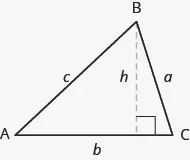### Example 9.33

Find the area of a triangle whose base is $1111$ inches and whose height is $88$ inches.

### Try It 9.65

Find the area of a triangle with base $1313$ inches and height $22$ inches.

### Try It 9.66

Find the area of a triangle with base $1414$ inches and height $77$ inches.

### Example 9.34

The perimeter of a triangular garden is $2424$ feet. The lengths of two sides are $44$ feet and $99$ feet. How long is the third side?

### Try It 9.67

The perimeter of a triangular garden is $2424$ feet. The lengths of two sides are $1818$ feet and $2222$ feet. How long is the third side?

### Try It 9.68

The lengths of two sides of a triangular window are $77$ feet and $55$ feet. The perimeter is $1818$ feet. How long is the third side?

### Example 9.35

The area of a triangular church window is $9090$ square meters. The base of the window is $1515$ meters. What is the window’s height?

### Try It 9.69

The area of a triangular painting is $126126$ square inches. The base is $1818$ inches. What is the height?

### Try It 9.70

A triangular tent door has an area of $1515$ square feet. The height is $55$ feet. What is the base?

#### Isosceles and Equilateral Triangles

Besides the right triangle, some other triangles have special names. A triangle with two sides of equal length is called an isosceles triangle. A triangle that has three sides of equal length is called an equilateral triangle. Figure 9.24 shows both types of triangles.

Figure 9.24 In an isosceles triangle, two sides have the same length, and the third side is the base. In an equilateral triangle, all three sides have the same length.

### Isosceles and Equilateral Triangles

An isosceles triangle has two sides the same length.

An equilateral triangle has three sides of equal length.

### Example 9.36

The perimeter of an equilateral triangle is $9393$ inches. Find the length of each side.

### Try It 9.71

Find the length of each side of an equilateral triangle with perimeter $3939$ inches.

### Try It 9.72

Find the length of each side of an equilateral triangle with perimeter $5151$ centimeters.

### Example 9.37

Arianna has $156156$ inches of beading to use as trim around a scarf. The scarf will be an isosceles triangle with a base of
$6060$ inches. How long can she make the two equal sides?

### Try It 9.73

A backyard deck is in the shape of an isosceles triangle with a base of $2020$ feet. The perimeter of the deck is $4848$ feet. How long is each of the equal sides of the deck?

### Try It 9.74

A boat’s sail is an isosceles triangle with base of $88$ meters. The perimeter is $2222$ meters. How long is each of the equal sides of the sail?

### Use the Properties of Trapezoids

A trapezoid is four-sided figure, a quadrilateral, with two sides that are parallel and two sides that are not. The parallel sides are called the bases. We call the length of the smaller base $b,b,$ and the length of the bigger base $B.B.$ The height, $h,h,$ of a trapezoid is the distance between the two bases as shown in Figure 9.25.

Figure 9.25 A trapezoid has a larger base, $B,B,$ and a smaller base, $b.b.$ The height $hh$ is the distance between the bases.

The formula for the area of a trapezoid is:

$Areatrapezoid=12h(b+B)Areatrapezoid=12h(b+B)$

Splitting the trapezoid into two triangles may help us understand the formula. The area of the trapezoid is the sum of the areas of the two triangles. See Figure 9.26.

Figure 9.26 Splitting a trapezoid into two triangles may help you understand the formula for its area.

The height of the trapezoid is also the height of each of the two triangles. See Figure 9.27.

Figure 9.27

The formula for the area of a trapezoid is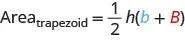If we distribute, we get,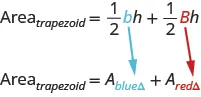### Properties of Trapezoids

• A trapezoid has four sides. See Figure 9.25.
• Two of its sides are parallel and two sides are not.
• The area, $A,A,$ of a trapezoid is $A=12h(b+B)A=12h(b+B)$.

### Example 9.38

Find the area of a trapezoid whose height is 6 inches and whose bases are $1414$ and $1111$ inches.

### Try It 9.75

The height of a trapezoid is $1414$ yards and the bases are $77$ and $1616$ yards. What is the area?

### Try It 9.76

The height of a trapezoid is $1818$ centimeters and the bases are $1717$ and $88$ centimeters. What is the area?

### Example 9.39

Find the area of a trapezoid whose height is $55$ feet and whose bases are $10.310.3$ and $13.713.7$ feet.

### Try It 9.77

The height of a trapezoid is $77$ centimeters and the bases are $4.64.6$ and $7.47.4$ centimeters. What is the area?

### Try It 9.78

The height of a trapezoid is $99$ meters and the bases are $6.26.2$ and $7.87.8$ meters. What is the area?

### Example 9.40

Vinny has a garden that is shaped like a trapezoid. The trapezoid has a height of $3.43.4$ yards and the bases are $8.28.2$ and $5.65.6$ yards. How many square yards will be available to plant?

### Try It 9.79

Lin wants to sod his lawn, which is shaped like a trapezoid. The bases are $10.810.8$ yards and $6.76.7$ yards, and the height is $4.64.6$ yards. How many square yards of sod does he need?

### Try It 9.80

Kira wants cover his patio with concrete pavers. If the patio is shaped like a trapezoid whose bases are $1818$ feet and $1414$ feet and whose height is $1515$ feet, how many square feet of pavers will he need?

### Section 9.4 Exercises

#### Practice Makes Perfect

Understand Linear, Square, and Cubic Measure

In the following exercises, determine whether you would measure each item using linear, square, or cubic units.

129.

amount of water in a fish tank

130.

length of dental floss

131.

living area of an apartment

132.

floor space of a bathroom tile

133.

height of a doorway

134.

capacity of a truck trailer

In the following exercises, find the perimeter and area of each figure. Assume each side of the square is $11$ cm.

135.136.137.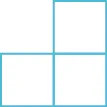138.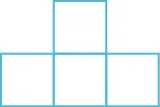139.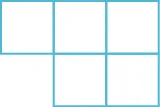140.Use the Properties of Rectangles

In the following exercises, find the perimeter and area of each rectangle.

141.

The length of a rectangle is $8585$ feet and the width is $4545$ feet.

142.

The length of a rectangle is $2626$ inches and the width is $5858$ inches.

143.

A rectangular room is $1515$ feet wide by $1414$ feet long.

144.

A driveway is in the shape of a rectangle $2020$ feet wide by $3535$ feet long.

In the following exercises, solve.

145.

Find the length of a rectangle with perimeter $124124$ inches and width $3838$ inches.

146.

Find the length of a rectangle with perimeter $20.220.2$ yards and width of $7.87.8$ yards.

147.

Find the width of a rectangle with perimeter $9292$ meters and length $1919$ meters.

148.

Find the width of a rectangle with perimeter $16.216.2$ meters and length $3.23.2$ meters.

149.

The area of a rectangle is $414414$ square meters. The length is $1818$ meters. What is the width?

150.

The area of a rectangle is $782782$ square centimeters. The width is $1717$ centimeters. What is the length?

151.

The length of a rectangle is $99$ inches more than the width. The perimeter is $4646$ inches. Find the length and the width.

152.

The width of a rectangle is $88$ inches more than the length. The perimeter is $5252$ inches. Find the length and the width.

153.

The perimeter of a rectangle is $5858$ meters. The width of the rectangle is $55$ meters less than the length. Find the length and the width of the rectangle.

154.

The perimeter of a rectangle is $6262$ feet. The width is $77$ feet less than the length. Find the length and the width.

155.

The width of the rectangle is $0.70.7$ meters less than the length. The perimeter of a rectangle is $52.652.6$ meters. Find the dimensions of the rectangle.

156.

The length of the rectangle is $1.11.1$ meters less than the width. The perimeter of a rectangle is $49.449.4$ meters. Find the dimensions of the rectangle.

157.

The perimeter of a rectangle of $150150$ feet. The length of the rectangle is twice the width. Find the length and width of the rectangle.

158.

The length of a rectangle is three times the width. The perimeter is $7272$ feet. Find the length and width of the rectangle.

159.

The length of a rectangle is $33$ meters less than twice the width. The perimeter is $3636$ meters. Find the length and width.

160.

The length of a rectangle is $55$ inches more than twice the width. The perimeter is $3434$ inches. Find the length and width.

161.

The width of a rectangular window is $2424$ inches. The area is $624624$ square inches. What is the length?

162.

The length of a rectangular poster is $2828$ inches. The area is $13161316$ square inches. What is the width?

163.

The area of a rectangular roof is $23102310$ square meters. The length is $4242$ meters. What is the width?

164.

The area of a rectangular tarp is $132132$ square feet. The width is $1212$ feet. What is the length?

165.

The perimeter of a rectangular courtyard is $160160$ feet. The length is $1010$ feet more than the width. Find the length and the width.

166.

The perimeter of a rectangular painting is $306306$ centimeters. The length is $1717$ centimeters more than the width. Find the length and the width.

167.

The width of a rectangular window is $4040$ inches less than the height. The perimeter of the doorway is $224224$ inches. Find the length and the width.

168.

The width of a rectangular playground is $77$ meters less than the length. The perimeter of the playground is $4646$ meters. Find the length and the width.

Use the Properties of Triangles

In the following exercises, solve using the properties of triangles.

169.

Find the area of a triangle with base $1212$ inches and height $55$ inches.

170.

Find the area of a triangle with base $4545$ centimeters and height $3030$ centimeters.

171.

Find the area of a triangle with base $8.38.3$ meters and height $6.16.1$ meters.

172.

Find the area of a triangle with base $24.224.2$ feet and height $20.520.5$ feet.

173.

A triangular flag has base of $11$ foot and height of $1.51.5$ feet. What is its area?

174.

A triangular window has base of $88$ feet and height of $66$ feet. What is its area?

175.

If a triangle has sides of $66$ feet and $99$ feet and the perimeter is $2323$ feet, how long is the third side?

176.

If a triangle has sides of $1414$ centimeters and $1818$ centimeters and the perimeter is $4949$ centimeters, how long is the third side?

177.

What is the base of a triangle with an area of $207207$ square inches and height of $1818$ inches?

178.

What is the height of a triangle with an area of $893893$ square inches and base of $3838$ inches?

179.

The perimeter of a triangular reflecting pool is $3636$ yards. The lengths of two sides are $1010$ yards and $1515$ yards. How long is the third side?

180.

A triangular courtyard has perimeter of $120120$ meters. The lengths of two sides are $3030$ meters and $5050$ meters. How long is the third side?

181.

An isosceles triangle has a base of $2020$ centimeters. If the perimeter is $7676$ centimeters, find the length of each of the other sides.

182.

An isosceles triangle has a base of $2525$ inches. If the perimeter is $9595$ inches, find the length of each of the other sides.

183.

Find the length of each side of an equilateral triangle with a perimeter of $5151$ yards.

184.

Find the length of each side of an equilateral triangle with a perimeter of $5454$ meters.

185.

The perimeter of an equilateral triangle is $1818$ meters. Find the length of each side.

186.

The perimeter of an equilateral triangle is $4242$ miles. Find the length of each side.

187.

The perimeter of an isosceles triangle is $4242$ feet. The length of the shortest side is $1212$ feet. Find the length of the other two sides.

188.

The perimeter of an isosceles triangle is $8383$ inches. The length of the shortest side is $2424$ inches. Find the length of the other two sides.

189.

A dish is in the shape of an equilateral triangle. Each side is $88$ inches long. Find the perimeter.

190.

A floor tile is in the shape of an equilateral triangle. Each side is $1.51.5$ feet long. Find the perimeter.

191.

A road sign in the shape of an isosceles triangle has a base of $3636$ inches. If the perimeter is $9191$ inches, find the length of each of the other sides.

192.

A scarf in the shape of an isosceles triangle has a base of $0.750.75$ meters. If the perimeter is $22$ meters, find the length of each of the other sides.

193.

The perimeter of a triangle is $3939$ feet. One side of the triangle is $11$ foot longer than the second side. The third side is $22$ feet longer than the second side. Find the length of each side.

194.

The perimeter of a triangle is $3535$ feet. One side of the triangle is $55$ feet longer than the second side. The third side is $33$ feet longer than the second side. Find the length of each side.

195.

One side of a triangle is twice the smallest side. The third side is $55$ feet more than the shortest side. The perimeter is $1717$ feet. Find the lengths of all three sides.

196.

One side of a triangle is three times the smallest side. The third side is $33$ feet more than the shortest side. The perimeter is $1313$ feet. Find the lengths of all three sides.

Use the Properties of Trapezoids

In the following exercises, solve using the properties of trapezoids.

197.

The height of a trapezoid is $1212$ feet and the bases are $99$ and $1515$ feet. What is the area?

198.

The height of a trapezoid is $2424$ yards and the bases are $1818$ and $3030$ yards. What is the area?

199.

Find the area of a trapezoid with a height of $5151$ meters and bases of $4343$ and $6767$ meters.

200.

Find the area of a trapezoid with a height of $6262$ inches and bases of $5858$ and $7575$ inches.

201.

The height of a trapezoid is $1515$ centimeters and the bases are $12.512.5$ and $18.318.3$ centimeters. What is the area?

202.

The height of a trapezoid is $4848$ feet and the bases are $38.638.6$ and $60.260.2$ feet. What is the area?

203.

Find the area of a trapezoid with a height of $4.24.2$ meters and bases of $8.18.1$ and $5.55.5$ meters.

204.

Find the area of a trapezoid with a height of $32.532.5$ centimeters and bases of $54.654.6$ and $41.441.4$ centimeters.

205.

Laurel is making a banner shaped like a trapezoid. The height of the banner is $33$ feet and the bases are $44$ and $55$ feet. What is the area of the banner?

206.

Niko wants to tile the floor of his bathroom. The floor is shaped like a trapezoid with width $55$ feet and lengths $55$ feet and $88$ feet. What is the area of the floor?

207.

Theresa needs a new top for her kitchen counter. The counter is shaped like a trapezoid with width $18.518.5$ inches and lengths $6262$ and $5050$ inches. What is the area of the counter?

208.

Elena is knitting a scarf. The scarf will be shaped like a trapezoid with width $88$ inches and lengths $48.248.2$ inches and $56.256.2$ inches. What is the area of the scarf?

#### Everyday Math

209.

Fence Jose just removed the children’s playset from his back yard to make room for a rectangular garden. He wants to put a fence around the garden to keep out the dog. He has a $5050$ foot roll of fence in his garage that he plans to use. To fit in the backyard, the width of the garden must be $1010$ feet. How long can he make the other side if he wants to use the entire roll of fence?

210.

Gardening Lupita wants to fence in her tomato garden. The garden is rectangular and the length is twice the width. It will take $4848$ feet of fencing to enclose the garden. Find the length and width of her garden.

211.

Fence Christa wants to put a fence around her triangular flowerbed. The sides of the flowerbed are $66$ feet, $88$ feet, and $1010$ feet. The fence costs $1010$ per foot. How much will it cost for Christa to fence in her flowerbed?

212.

Painting Caleb wants to paint one wall of his attic. The wall is shaped like a trapezoid with height $88$ feet and bases $2020$ feet and $1212$ feet. The cost of the painting one square foot of wall is about $0.05.0.05.$ About how much will it cost for Caleb to paint the attic wall?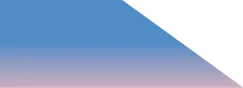#### Writing Exercises

213.

If you need to put tile on your kitchen floor, do you need to know the perimeter or the area of the kitchen? Explain your reasoning.

214.

If you need to put a fence around your backyard, do you need to know the perimeter or the area of the backyard? Explain your reasoning.

215.

Look at the two figures.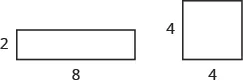Which figure looks like it has the larger area? Which looks like it has the larger perimeter?

Now calculate the area and perimeter of each figure. Which has the larger area? Which has the larger perimeter?

216.

The length of a rectangle is $55$ feet more than the width. The area is $5050$ square feet. Find the length and the width.

Write the equation you would use to solve the problem.

Why can’t you solve this equation with the methods you learned in the previous chapter?

#### Self Check

After completing the exercises, use this checklist to evaluate your mastery of the objectives of this section.

On a scale of 1–10, how would you rate your mastery of this section in light of your responses on the checklist? How can you improve this?

Order a print copy

As an Amazon Associate we earn from qualifying purchases.